Integral with summations inside

I am a big fan of blackpenredpen. This guy posts videos on Youtube about calculus and more. Some days ago I received a notification and this is what the thumbnail contained:I thought this was just insane and I had no idea how to start, but I gave it a try anyway. You can click here for his video!

Although you might be tempted, you can’t simplify x^k because that’s a sum. Instead, let’s expand this to see if there’s any pattern. First though, let’s take the integral of the first term, which is way easier (we’re applying linearity): we know that the antiderivative of 1/x is ln(x), therefore the integral of 1/(x-1) will be ln(x-1). At this point we can write: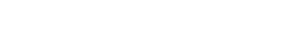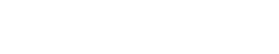Now, let’s analyse each sum individually:This is simply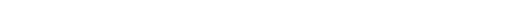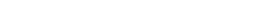This looks a lot like a sum of derivatives, to be more specific the sum of the first derivative of x^k: the derivative of x is 1, of x² is 2x, of x³ is 3x² and so on. But there’s nothing we can do right now. Let’s give a look at the other sum: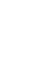We get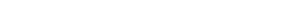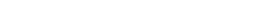Here instead we have the sum of the function x^k, and what’s cool is that this sum is actually the derivative of the first sum, as we saw before! This means we can perform a u-substitution by letting u be equal to this sum so that its derivative will cancel out with the other sum. First though, I would like to write the integral in a different way:This was just to make things less messy. At this point we can substitute: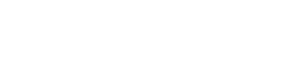Notice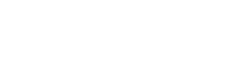The part in blue is our du and the one in red is our u; this becomes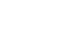I couldn’t believe it all reduced to this way too easy integral, and I didn’t know whether to be excited or disappointed because it thought it could not be possible, but I kept going. So, as we know, the antiderivative of this is simply ln(u), and so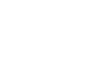Let’s not forget about the first integral though! The two combined give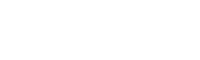Now, this is a sum of two logarithms: this is one of the properties: ln(a)+ln(b)=ln(a*b), therefore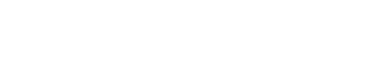Let’s now see what we get from each sum: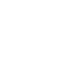This is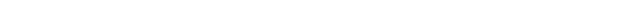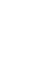As we saw before, this isNow, what happens when we subtract them? (this is what’s inside ln, so we need to solve for it):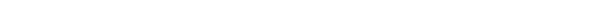You can see it better here: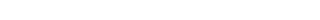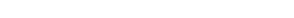As you can see, each term cancels out except for the first term, -1 and the last term, +x^2020. This simplifies amazingly!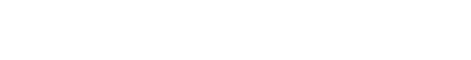When I checked for the answer at the end of the video, I was so happy!

Finally, we have: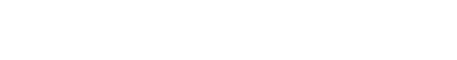How satisfying is this? I just loved it!